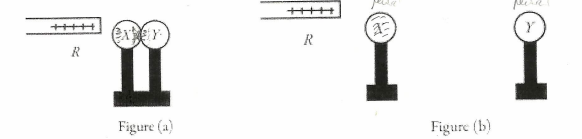# Problem: X and Y are two uncharged metal spheres on insulating stands, and are in contact with each other. A positively charged rod R is brought close to X as shown in Figure (a). Sphere Y is now moved away from X, as in Figure (b). What are the final charge states of X and Y?(a) X is positive and Y is neutral.(b) Both X and Y are neutral.(c) X is negative and Y is positive.(d) Both X and Y are negative.(e) X is neutral and Y is positive.

###### FREE Expert Solution
82% (483 ratings)
###### Problem Details

X and Y are two uncharged metal spheres on insulating stands, and are in contact with each other. A positively charged rod R is brought close to X as shown in Figure (a). Sphere Y is now moved away from X, as in Figure (b). What are the final charge states of X and Y?

(a) X is positive and Y is neutral.

(b) Both X and Y are neutral.

(c) X is negative and Y is positive.

(d) Both X and Y are negative.

(e) X is neutral and Y is positive.Frequently Asked Questions

What scientific concept do you need to know in order to solve this problem?

Our tutors have indicated that to solve this problem you will need to apply the Charging Objects concept. You can view video lessons to learn Charging Objects. Or if you need more Charging Objects practice, you can also practice Charging Objects practice problems.

What professor is this problem relevant for?

Based on our data, we think this problem is relevant for Professor Jerousek's class at UCF.